Altair® Panopticon

# Slope

The slope of the least-squares line.

The formula: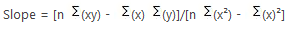Sample 1:

Give the set of X and Y values where X and Y can represent any correlated values below:

 X Y 1 2 2 4 3 6 4 8 5 10 6 12 7 14 8 16 9 18 10 20

Sample table

## Computing the Slope

1. Solve the parts of the formula: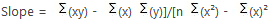n =count of items, equal to 10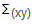= multiply all x and y items and get the sum = 770

1x2 + 2x4 + 3x6 + 4x8 + 5x10 + 6x12 + 7x14 + 8x16 + 9x18 + 10x20 = 770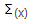= sum of x items = 55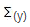= sum of y items = 110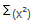= get the square of all x items and sum up the values. To square a number also means to multiply the number by itself.

1x1 + 2x2 + 3x3 + 4x4 + 5x5 + 6x6 + 7x7 + 8x8 + 9x9 + 10x10 = 385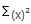= get the sum of all items in x and get the square = 55 * 55 or 3025

1. Substitute the known values in the formula and computed for the Slope: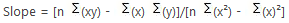Slope = [10(770) – 55(110)]/[10(385) – 3025]

Slope = [7700 – 6050]/3850-3025]

Slope = 1650/825

Slope = 2